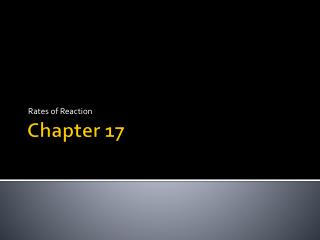# Chapter 17 - PowerPoint PPT PresentationDownload PresentationChapter 17

Chapter 17Download Presentation## Chapter 17

- - - - - - - - - - - - - - - - - - - - - - - - - - - E N D - - - - - - - - - - - - - - - - - - - - - - - - - - -
##### Presentation Transcript

1. Rates of Reaction Chapter 17

2. Rate of Reaction Rate of Reaction = change in concentration Time for change to occur Change or concentration can be for reactant or product

3. Finding the Average Reaction Rate Rate of Reaction = 0.100M – 0.220M 4.00s – 0.00s Rate of Reaction = 0.0300 M/s

4. Collision Theory Collision Theory states that atoms, molecules and ions must collide to react • A collision must occur to react • Molecule must have the correct orientation to collide with enough activation energy to start the reaction • Collision must have enough energy to form an activated complex

5. Orientation and Activated Complex CO(g) + NO2(g)  CO2(g) + NO(g) Carbon from CO must contact Oxygen on impact from NO2 in order for the reaction to occur The impact must provide enough energy that the collision forms an activated complex Activated Complex: temporary unstable arrangement of atoms that may from products or break apart to re-form reactants. (Transition State)

6. Image 17-1 CO(g) + NO2(g)  CO2(g) + NO(g)

7. Activation Energy and Reactions • Activation Energy Ea – the minimum amount of energy required by reactants to form an activated complex leading to a reaction • Activation Energy influences rate of reaction • High Ea - few collisions produce enough E, reaction rate low • Low Ea – many collisions produce enough E, reaction rate higher

8. Energy Diagram

9. Energy Diagram CO and NO2

10. Rate of Reaction and Spontaneity Spontaneity related to Free Energy (ΔG) and Enthalpy (ΔH) • If ΔG negative reaction spontaneous • If ΔG positive not spontaneous • If ΔH positive reaction endothermic • If ΔH negative reaction exothermic Spontaneity does not affect Rate of Reaction

11. Factors Affecting Rate of Reaction • 1. Nature of Reactants • 2. Concentration • 3. Surface Area • 4. Temperature • 5. Catalysts

12. Nature of Reactants • How likely is the substance to react? • Metals Ca and Na both react in H2O • Slow to form H2 gas (Ca less reactive) Ca(s) + 2H2O(l)  H2(g) + Ca(OH)2 (aq) • Fast to form H2 gas (Na more reactive) 2Na(s) + 2H2O(l)  H2(g) + 2NaOH (aq)

13. Concentration • As concentration (or reactants) increases, Rate of reaction increases • Increase Concentration increases number of collisions

14. Surface Area • Greater surface area leads to increased rate of reaction • The larger the surface area, the more collisions can occur with the substance • Solid Steel vs. Steel Wool • Pulverizing or grinding a substance will increase it’s surface area

15. Temperature • Increasing temperature increases rate of reaction (for most reactions) • Increasing temp increases KE • Increasing KE increases collisions • Increasing KE also increases the intensity of the collisions with more of them able to overcome the activation E • Increasing T is not always applicable

16. Catalysts • Catalyst is a substance that increases the rate of reaction without being consumed in the reaction • Catalyst is not included in chemical equation • Catalyst does not increase amount of product formed • Catalyst decreases the activation E due to more collisions having sufficient E for the reaction • Ex: Enzyme

17. Catalysts (Con’t) • Inhibitors are the reverse of catalyst • Slow down the reaction time. Used for food preservatives • Heterogeneous Catalyst – physical state is different then reaction it catalyzes ahhhh • Catalytic converter uses transition metal catalyst as solid in gaseous reaction • Homogeneous Catalyst – same physical state daddy • Both enzyme and reaction are in aqueous sol’n

18. Reaction Rate Laws • Rate Law: expresses the mathematical relationship in a reaction • Reaction Order: for a reactant defines how it is affected by concentrations of that reactant • Specific Rate Constant: k is the numerical value that relates to that reaction rate and concentration of reactants at specific T (L/mol ·s) • k changes with Temp • Larger k, faster reaction • Smaller k , slower reaction

19. Reaction Order First Order Reaction A  B The rate of reaction depends directly on the concentration of A Rate = k[A] Other Order Reactions depends on sum of reaction orders for individual reactants aA + bB products Rate = k [A]m[B]n If reaction is single step then m =a and n=b

20. Determining Reaction Order • Method of Initial Rate: compare initial rate rxn carried out with varied concentration of reactants • aA + bB products • Rate = k [A]1 [B]2 overall third order (2+1)

21. Instantaneous Rate • The rate of decomposition at a specific time • Can be found by using slope or by experimentally determined rate law ahh daddy

22. Sample Problem 2 NO + H2 N2O + H2O First order H2 second order NO K = 2.90x102 L2/mol2 s Find the instantaneous rate when [NO] = 0.00500M and [H2] = 0.00200M

23. Reaction Mechanisms • Reaction mechanism is the series of steps that make up a complex reaction • Complex reaction is a reaction with two or more elementary steps • By adding the reactions and cancelling out the formulas that occur on both sides of the equation you get the net reaction • Intermediates are substances produced in one elementary step and consumed in another elementary step

24. Rate Determining Step • The slowest step in the reaction determines the rate for the reaction